Lakhmir Singh & Manjit Kaur: Chemical Reactions and Equations, Solutions- 4

# Lakhmir Singh & Manjit Kaur: Chemical Reactions and Equations, Solutions- 4 - Class 10 Chemistry Solutions By Lakhmir Singh & Manjit Kaur

(Page No - 47)

Question 27:
(a) Define a combination reaction.
(b) Give one example of a combination reaction that is also exothermic.
(c) Give one example of a combination reaction that is also endothermic.
Solution :

(a) Those reactions in which two or more substances combine to form a single substance is called
a combination reaction.

Question 28:
(a) Give an example of an oxidation reaction.
(b) Is oxidation an exothermic or an endothermic reaction?
(c) Explain, by giving an example, how oxidation and reduction proceed side by side.
Solution :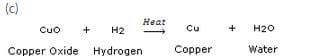In this reaction, copper oxide is being reduced to copper whereas hydrogen is being oxidised to water.

Question 29:
(a) What is the colour of ferrous sulphate crystals ? How does this colour change   after heating ?
(b) Name the product formed on strongly heating ferrous sulphate crystals. What type of chemical reaction
occurs in this change ?
Solution :

(a) The colour of ferrous sulphate is green. It changes to brown after heating.
(b) The product formed is ferric oxide. This is a decomposition reaction.

Question 30:
What is a decomposition reaction ? Give an example of a decomposition reaction. Describe an activity to illustrate such a reaction by heating.
Solution :

Those reactions in which a compound splits up into tvvo or more simpler substances is called a decomposition reaction.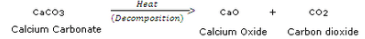When calcium carbonate is heated, it decomposes to give calcium oxide and carbon dioxide.
Activity: When potassium chlorrate is heated in the presence of manganese dioxide catalyst, it decomposes to give potassium chloride and Oxygen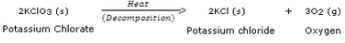This decomposition takes place in the presence of heat and catalyst. In this decomposition reaction, a single compound, potassium chlorate, is splitting up into two simpler substances, potassium chloride and oxygen. This decomposition reaction is used for preparing oxygen gas in the laboratory.

Question 31:
Zinc oxide reacts with carbon, on heating, to form zinc metal and carbon monoxide. Write a balanced chemical equation for this reaction. Name (i) oxidising agent, and (ii) reducing agent, in this reaction.
Solution :

ZnO + C
(i) Zinc oxide.
(ii) Carbon.

Question 32:
Give one example of an oxidation-reduction reaction which is also :
(a) a combination reaction
(b) a displacement reaction
Solution :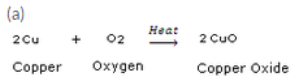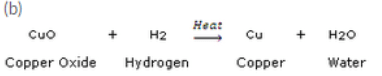Question 33:
(a) What is the difference between displacement and double displacement reactions ? Write equations for
these reactions.
(b) What do you mean by a precipitation reaction ? Explain giving an example.
Solution :

(a) Those reactions in which one element takes the place of another element in a compound, are known as displacement reactions.
Equation: CuSO4 (aq)+ Zn (s)—–>ZnSO4+ Cu
Those reactions in which two compounds react by an exchange of ions to form two new compounds are called double displacement reactions.
Equation: AgNO3 (aq) + NaCl (aq)—–>AgCl (s)+ NaNO3 (aq)
(b) Any reaction in which an insoluble solid (called precipitate) is formed that separates from the solution is called a precipitation reaction.
Example: The reaction between barium chloride and sodium sulphate solution to form a white barium sulphate precipitate (alongwith sodium chloride solution) is an example of a precipitation reaction.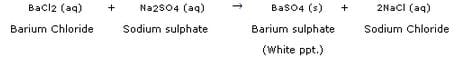Question 35:
(a) Define the following in terms of gain or loss of hydrogen with one example each :
(i) oxidation (ii) reduction
(b) When a magnesium ribbon is heated, it burns in air to form magnesium oxide. Write a balanced chemical equation for this reaction. Name (i) substance oxidised, and (ii) substance reduced.
Solution :

(a) (i) H2S + Cl2—–>S + 2HCl
In this reaction, H2S is changing into S. That is, Hydrogen is being removed from hydrogen sulphide. Now, by definition, the removal of hydrogen from a compound is called oxidation, so, we can say that hydrogen sulphide is being oxidised to sulphur. On the other hand, Cl2 is changing into HCl. That is, hydrogen is being added to chlorine. By definition, the addition of hydrogen to a substance is called reduction, so we can say that chlorine is being reduced to hydrogen chloride.
(b) 2Mg + O2—–>2MgO
(i) Mg (ii) O2

Question 36:
What is meant by (a) displacement reaction, and (b) double displacement reaction ? Explain with the help of one example each.
Solution :

(a) Those reactions in which one element takes the place of another element in a compound, are known as displacement reactions.
Example: CuSO4 (aq) + Zn (s) —–>ZnSO4 + Cu
When a strip of zinc metal is placed in copper sulphate solution, then zinc sulphate solution and copper are obtained. In this reaction, zinc displaces copper from copper sulphate compound so that copper is set free. The blue colour of copper sulphate solution fades due to the formation of zinc sulphate.
(b) Those reactions in which two compounds react by an exchange of ions to form two new compounds are called double displacement reactions.
Example: AgNO3 (aq) + NaCl (aq)
When silver nitrate solution is added to sodium chloride solution, then a white precipitate of silver chloride is formed along with sodium nitrate solution. In this reaction, two compounds, silver nitrate and sodium chloride, react to form two new compounds, silver chloride and sodium nitrate.

Question 37:
(a) Why are decomposition reactions called the opposite of combination reactions ? Explain with equations
of these reactions.
(b) Express the following facts in the form of a balanced chemical equation :
“When a strip of copper metal is placed in a solution of silver nitrate, metallic silver is precipitated and a solution containing copper nitrate is formed”.
Solution :

Those reactions in which a compound splits up into two or more simpler substances are known as decomposition reactions.
Example: When calcium carbonate is heated, it decomposes to give calcium oxide and carbon dioxide.
They are called opposite of combination reactions because in a combination reaction, two or more substances combine to form a single substance.
Example: Magnesium and oxygen combine, when heated, to form magnesium oxide.
(b) Cu (s) + 2AgNO3 (aq)—–>Cu(NO3)2 (aq) + 2Ag (s)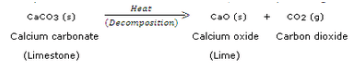They are called opposite of combination reactions because in a combination reaction, two or more substances combine to form a single substance.

Example: Magnesium and oxygen combine, when heated, to form magnesium oxide.

2Mg (s) Magnesium + 02 oxygen → 2Mg0 (s) Magnesium Oxide

Question 38:
(a) What happens when a piece of iron metal is placed in copper sulphate solution ? Name the type ofreaction involved.
(b) Write balanced chemical equation with state symbols for the following reaction :Barium chloride solution reacts with sodium sulphate solution to give insoluble barium sulphate and a solution of sodium chloride.
Solution :

(a) When a piece of iron metal is placed in copper sulphate solution, then magnesium sulphate solution and copper metal are formed. This is a type of displacement reaction.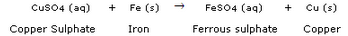(b)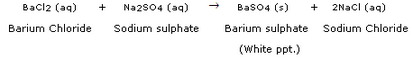Question 39:
In the reaction represented by the following equation :
CuO(s) + H2(g) ————- > Cu (s) + H20(1)
(a) name the substance oxidised
(b) name the substance reduced
(c) name the oxidising agent
(d) name the reducing agent
Solution :

(a) H2
(b) CuO
(c) CuO
(d) H2

Question 40:
What happens when silver nitrate solution is added to sodium chloride solution ?
(a) Write the equation for the reaction which takes place.
(b) Name the type of reaction involved.
Solution :

When silver nitrate
solution is added to sodium chloride solution, then a white precipitate of
silver chloride is formed alongwith sodium nitrate solution.
(a) AgNO3(aq) + NaCl (aq)
(b) Double displacement reaction.

Question 41:
What happens when silver chloride is exposed to sunlight ? Write a chemical equation for this reaction. Also give one use of such a reaction.
Solution :

When silver chloride is exposed to light, it decomposes to form silver metal and chlorine gas.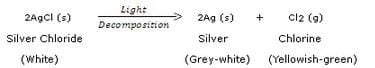This reaction is used in black and white photography.

Question 42:
What happens when a zinc strip is dipped into a copper sulphate solution ?
(a) Write the equation for the reaction that takes place.
(b) Name the type of reaction involved.
Solution :

When a strip of zinc metal is placed in copper sulphate solution, then zinc sulphate solution and copper are obtained.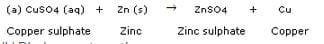(Page No - 48)

Question 43:
(a) Explain the term “corrosion” with an example. Write a chemical equation to show the process of corrosion of iron.
(b) What special name is given to the corrosion of iron ?
(c) What type of chemical reaction is involved in the corrosion of iron ?
(d) Name any three objects (or structures) which are gradually damaged by the corrosion of iron and steel.
Solution :

(a) Corrosion is the process in which metals are eaten up gradually by the action of air, moisture or a chemical (such as an acid) on their surface. Rusting of iron metal is the most common form of corrosion.
During the corrosion of iron, iron metal is oxidised by the oxygen of air in the presence of water (moisture) to form hydrated iron oxide called rust.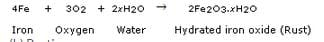(b) Rusting.
(c) Oxidation.
(d) Railings, car bodies and bridges.

Question 44:
(a) Explain the term “rancidity”. What damage is caused by rancidity ?
(b) What type of chemical reaction is responsible for causing rancidity ?
(c) State and explain the various methods for preventing or retarding rancidity of food.
Solution :

(a) The condition produced by aerial oxidation of fats and oils in foods marked by unpleasant smell and taste is called rancidity. It spoils the food materials prepared in fats and oils which have been kept for a considerable time and makes them unfit for eating.
(b) Oxidation.
(c) Rancidity can be prevented by adding anti-oxidants to foods containing fats and oils.

1. It can be prevented by packaging fat and oil containing foods in nitrogen gas.
2. Rancidity can be retarded by keeping food in a refrigerator.
3. Rancidity can be prevented by storing food in air-tight containers.
4. Storing foods away from light can also prevent rancidity.

Question 45:
(a) What happens when an aqueous solution of sodium sulphate reacts with an aqueous solution of barium chloride ?
(b) Write the balanced chemical equation for the reaction which takes place.
(c) State the physical conditions of reactants in which the reaction will not take place.
(d) Name the type of chemical reaction which occurs.
(e) Give one example of another reaction which is of the same type as the above reaction.
Solution :

(a) When barium chloride solution is added to sodium sulphate solution, then a white precipitate of barium sulphate is formed along with sodium chloride solution.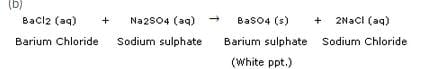(c) Solid sodium sulphate and Solid barium chloride
(d) Double displacement reaction.
(e) Double displacement reaction between silver nitrate solution and sodium chloride solution forms a white precipitate of silver chloride and sodium nitrate solution.

(Page No - 49)

Question 58:
When a green iron salt is heated strongly, its colour finally changes to brown and odour of burning sulphur is given out.
(a) Name the iron salt.
(b) Name the type of reaction that takes place during the heating of iron salt.
(c) Write a chemical equation for the reaction involved.
Solution :

(a) Ferrous sulphate.
(b) Decomposition reaction.
(c)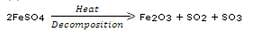Question 59:
A colourless lead salt, when heated, produces a yellow residue and brown fumes.
(b) Name the brown fumes.
(c) Write a chemical equation of the reaction involved.
Solution :

(b) Nitrogen dioxide.
(c)Question 60:
When hydrogen burns in oxygen, water is formed and when water is electrolysed, then hydrogen and oxygen are produced. What type of reaction takes place :
(a) in the first case ?
(b) in the second case ?
Solution :

(a) Combination reaction.
(b) Decomposition reaction.

Question 61:
A strip of metal X is dipped in a blue coloured salt solution YSO4. After some time, a layer of metal Y from the salt solution is formed on the surface of metal strip X. Metal X is used in galvanisation whereas metal Y is used in making electric wires. Metal X and metal Y together form an alloy Z.
(a) What could metal X be ?
(b) What could metal Y be ?
(c) Name the metal salt YSO4.
(d) What type of chemical reaction takes place when metal X reacts with salt solution YSO4 ? Write the equation of the chemical reaction involved.
(e) Name the alloy Z.
Solution :

(b) Copper (Cu)
(c) Copper sulphate (CuSO4)
(d) Displacement reaction;
CuSO(aq) + Zn (s)
ZnSO4 (aq) + Cu (s)
(e) Brass

Question 62:
When a black metal compound XO is heated with a colourless gas Y2, then metal X and another compound Y2O are formed. Metal X is red-brown in colour which does not react with dilute acids at all. Gas Y2 can be prepared by the action of a dilute acid on any active metal. The compound Y2O is a liquid at room temperature which can turn anhydrous copper sulphate blue.
(a) What do you think is metal X ?
(b) What could be gas Y2 ?
(c) What is compound XO ?
(d) What is compound Y2O?
(e) Write the chemical equation of the reaction which takes place on heating XO with Y2.
(f) What type of chemical reaction is illustrated in the above equation ?
Solution :

(a) Copper (Cu)
(b) Hydrogen (H2)
(c) Copper oxide (CuO)
(d) Water (H2O)
(e) CuO + H2
Cu + H2O
(f) Displacement reaction (which is also a redox reaction).

Question 63:
A metal X forms a water soluble salt XNO3 . When an aqueous solution of XNO3 is added to common salt solution, then a white precipitate of compound Y is formed along with sodium nitrate solution. Metal X is said to be the best conductor of electricity and it does not evolve hydrogen when put in dilute hydrohloric acid.
(a) What is metal X ?
(b) What is salt XNO3 ?
(c) Name the compound Y.
(d) Write the chemical equation of the reaction which takes place on reacting XNO3 solution and common salt solution giving the physical states of all the reactants and products.
(e) What type of chemical reaction is illustrated by the above equation ?
Solution :

Silver (Ag)
(b) Silver nitrate (AgNO3)
(c) Silver chloride (AgCl)
(d) AgNO3(aq) + NaCl (aq)—–>AgCl (s) + NaNO3 (aq)
(e) Double displacement reaction.

(Page No - 50)

Question 64:
Two metals X and Y form the salts XSO4 and Y2SO4, respectively. The solution of salt XSO4 is blue in colour whereas that of Y2SO4 is colourless. When barium chloride solution is added to XSO4 solution, then a white precipitate Z is formed along with a salt which turns the solution green. And when barium chloride solution is added to Y2SO4 solution, then the same white precipitate Z is formed along with colourless common salt solution.
(a) What could the metals X and Y be ?
(b) Write the name and formula of salt XSO4.
(c) Write the name and formula of salt Y2SO4.
(d) What is the name and formula of white precipitate Z ?
(e) Write the name and formula of the salt which turns the solution green in the first case.
Solution :

(a) Metal X : Copper; Metal Y : Sodium
(b) Copper sulphate, CuSO4
(c) Sodium sulphate, Na2SO4
(d) Barium sulphate, BaSO4
(e) Copper chloride, CuCl2

Question 65:
A red-brown metal X forms a salt XSO4. When hydrogen sulphide gas is passed through an aqueous solution of XSO4, then a black precipitate of XS is formed alongwith sulphuric acid solution.
(a) What could the salt XSO4 be ?
(b) What is the colour of salt XSO4 ?
(c) Name the black precipitate XS.
(d) By using the formula of the salt obtained in (a) above, write an equation of the reaction which takes place when hydrogen sulphide gas is passed through its aqueous solution.
(e) What type of chemical reaction takes place in this case ?
Solution :

(a) Copper sulphate.
(b) Blue colour.
(c) Copper sulphide.
(d) CuSO4 (aq) + H2S (g)—–>CuS (s) + H2SO(aq)
(e) Double displacement reaction.

Question 66:
When a strip of red-brown metal X is placed in a colourless salt solution YNOthen metal Y is set free and a blue coloured salt solution X(NO3)2 is formed. The liberated metal Y forms a shining white deposit on the strip of metal X.
(a) What do you think metal X is ?
(b) Name the salt YNO3.
(c) What could be metal Y ?
(d) Name the salt X(NO3)2.
(e) What type of reaction takes place between metal X and salt solution YNO3 ?
Solution :

(a) Copper.
(b) Silver nitrate.
(c) Silver.
(d) Copper nitrate.
(e) Displacement reaction.

Question 67:
A metal salt MX when exposed to light splits up to form metal M and a gas X2. Metal M is used in making ornaments whereas gas X2 is used in making bleaching powder. The salt MX is itself used in black and white photography.
(a) What do you think metal M is ?
(b) What could be gas X2 ?
(c) Name the metal salt MX.
(d) Name any two salt solutions which on mixing together can produce a precipitate of salt MX.
(e) What type of chemical reaction takes place when salt MX is exposed to light ? Write the equation of the reaction.
Solution :

(a) Silver.
(b) Chlorine.
(c) Silver chloride.
(d) Silver nitrate and Sodium chloride.
(e) Decomposition reaction;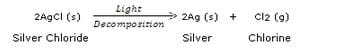The document Lakhmir Singh & Manjit Kaur: Chemical Reactions and Equations, Solutions- 4 | Class 10 Chemistry Solutions By Lakhmir Singh & Manjit Kaur is a part of the Class 10 Course Class 10 Chemistry Solutions By Lakhmir Singh & Manjit Kaur.
All you need of Class 10 at this link: Class 10

20 docs

## FAQs on Lakhmir Singh & Manjit Kaur: Chemical Reactions and Equations, Solutions- 4 - Class 10 Chemistry Solutions By Lakhmir Singh & Manjit Kaur

 1. What is a chemical reaction?Ans. A chemical reaction is a process in which one or more substances are converted into different substances with different properties. It involves the breaking and forming of chemical bonds to create new compounds.
 2. How can chemical reactions be represented?Ans. Chemical reactions can be represented using chemical equations. In a chemical equation, the reactants are written on the left side of the arrow and the products are written on the right side. The number of atoms of each element must be balanced on both sides of the equation.
 3. What is the importance of balancing chemical equations?Ans. Balancing chemical equations is important because it ensures that the law of conservation of mass is followed. According to this law, matter cannot be created or destroyed in a chemical reaction, only rearranged. Balancing the equations helps us to accurately represent the quantities of reactants and products involved in a reaction.
 4. How do we identify the type of chemical reaction?Ans. There are several types of chemical reactions, including synthesis, decomposition, combustion, displacement, and double displacement reactions. The type of reaction can be identified by analyzing the reactants and products involved. For example, a synthesis reaction involves the combination of two or more substances to form a single product, while a decomposition reaction involves the breakdown of a compound into simpler substances.
 5. How can we predict the products of a chemical reaction?Ans. Predicting the products of a chemical reaction requires a good understanding of the types of reactions and the reactivity of different substances. By analyzing the reactants and their properties, we can make educated guesses about the products that will be formed. However, experimental evidence and knowledge of chemical principles are often necessary to accurately predict the products.

## Class 10 Chemistry Solutions By Lakhmir Singh & Manjit Kaur

20 docsExplore Courses for Class 10 examSignup to see your scores go up within 7 days! Learn & Practice with 1000+ FREE Notes, Videos & Tests.
10M+ students study on EduRev
Track your progress, build streaks, highlight & save important lessons and more!
Related Searches

,

,

,

,

,

,

,

,

,

,

,

,

,

,

,

,

,

,

,

,

,

,

,

,

;